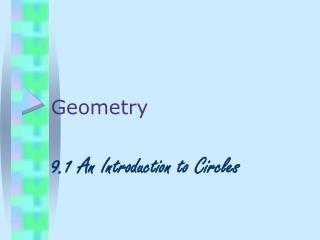DownloadDownload PresentationGeometry

# Geometry

Download Presentation## Geometry

- - - - - - - - - - - - - - - - - - - - - - - - - - - E N D - - - - - - - - - - - - - - - - - - - - - - - - - - -
##### Presentation Transcript

1. Geometry 9.1 An Introduction to Circles

2. Circle • The set of all points in a plane at a given • distance from a given point. . P 3 P is the center of the circle. The circle is the set of all points in the plane of the screen 3 away from P.

3. Radius Plural is radii • A segment that joins the center of the • circle to any point on the circle. . P radius

4. Chord • A segment whose endpoints lie on a circle. . P chord

5. Diameter • A chord that passes through the center • of the circle. twice The diameter is _________ as long as the radius. . P diameter

6. Secant • A line that contains a chord of a circle. . P SECANT

7. Tangent • A line in the plane of a circle that • intersects the circle in exactly one point. . P Tangent . Point of tangency

8. F B E A C D Name: 1) The center: A

9. F B E A C D Name: 2) Two diameters: DB and FC

10. F B E A C D Name: 3) A point of tangency: D

11. F B E A C D Name: 4) Four radii: AB and AF and AC AD and

12. F B E A C D Name: 5) A tangent: ED

13. F B E A C D Name: 6) A secant: FC

14. F B E A C D Name: 7) Six chords: FB and and DF DC and and and BC DB FC

15. F B E A C D 8) Why is AC not a Chord of A: . A chord is a segment with both endpoints on the circle. AC has only one endpoint on the circle.

16. F B E A C D 9) Why is BD not a Chord of A: . A chord is a segment not a line.

17. G F H L I X K J Sphere • Sphere: The set of all points in space given distance from any given point is a sphere. • Many of the terms used with circles are also used with spheres. • For example, sphere X has a… center: radii: chords: diameter: secants: tangent: point of tangency:

18. 4 4 Congruent Circles congruent circles/spheres Circles (or spheres) are congruent if they have congruent radii.

19. Concentric Circles concentric circles: Circles that lie in the same plane and have the same center are concentric. concentric spheres: Concentric spheres have the same center.

20. Inscribed Polygon • A polygon is inscribed in a circle if each vertex of the polygon lies on the circle. • A circle is circumscribed about a polygon if each vertex of the polygon lies on the circle. The polygon is inscribed in the circle. Thus, the circle is circumscribed about the polygon. These two sentences have the same meaning.

21. A F C G D In sphere A, draw: 10. a diameter, BC 11. a chord, DE 12. a tangent, CF 13. a secant, DG B C E D

22. 14. Draw two concentric circles. Draw a tangent to one of the circles. Is it tangent to the other circle? • 15. Draw a large circle. Inscribe an isosceles triangle in the circle. • 16. Draw a rectangle. Circumscribe a circle about the rectangle. No.

23. O 6 x B Find the value of x. 6 6 60 3 90 3√3 30 3√3 3√3 x = 6√3

24. x 8 60 O x O 3 4 4 O x Find the value of x. O is the center of each circle. x = 8 x = 5 x x = 4√2 x 10 45 O x = 5√2

25. HW • P. 330-340 CE 1-11 WE 1-15